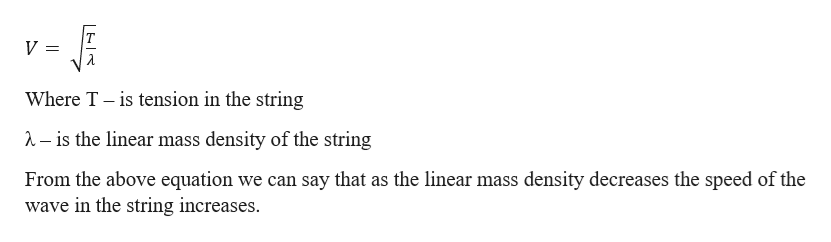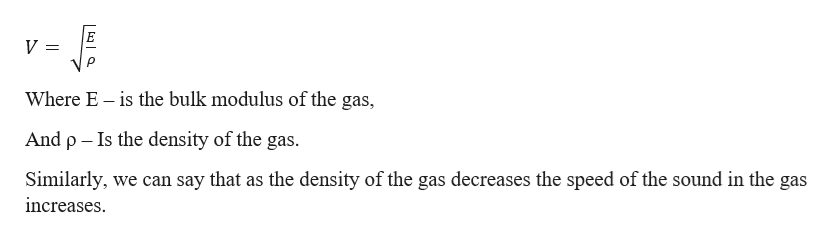# How does the speed of sound increasing when the temperature increases be explained using a parallel to the speed of a wave on a string? Since the wave on a string is transverse and only depends on tension and mass density of the medium, how would this be relevant to the speed of sound (which is longitudinal?)

Question

How does the speed of sound increasing when the temperature increases be explained using a parallel to the speed of a wave on a string? Since the wave on a string is transverse and only depends on tension and mass density of the medium, how would this be relevant to the speed of sound (which is longitudinal?)

check_circleExpert Solution
Step 1

Speed of transverse wave in a string is given by the equation:help_outlineImage TranscriptioncloseWhere T is tension in the string -is the linear mass density of the string From the above equation we can say that as the linear mass density decreases the speed of the wave in the string increases. fullscreen
Step 2

The speed of sound in a ...help_outlineImage Transcriptionclose= Where E is the bulk modulus of the gas, And p Is the density of the gas. Similarly, we can say that as the density of the gas decreases the speed of the sound in the gas increases fullscreen

### Want to see the full answer?

See Solution

#### Want to see this answer and more?

Solutions are written by subject experts who are available 24/7. Questions are typically answered within 1 hour*

See Solution
*Response times may vary by subject and question
Tagged in

### Physics